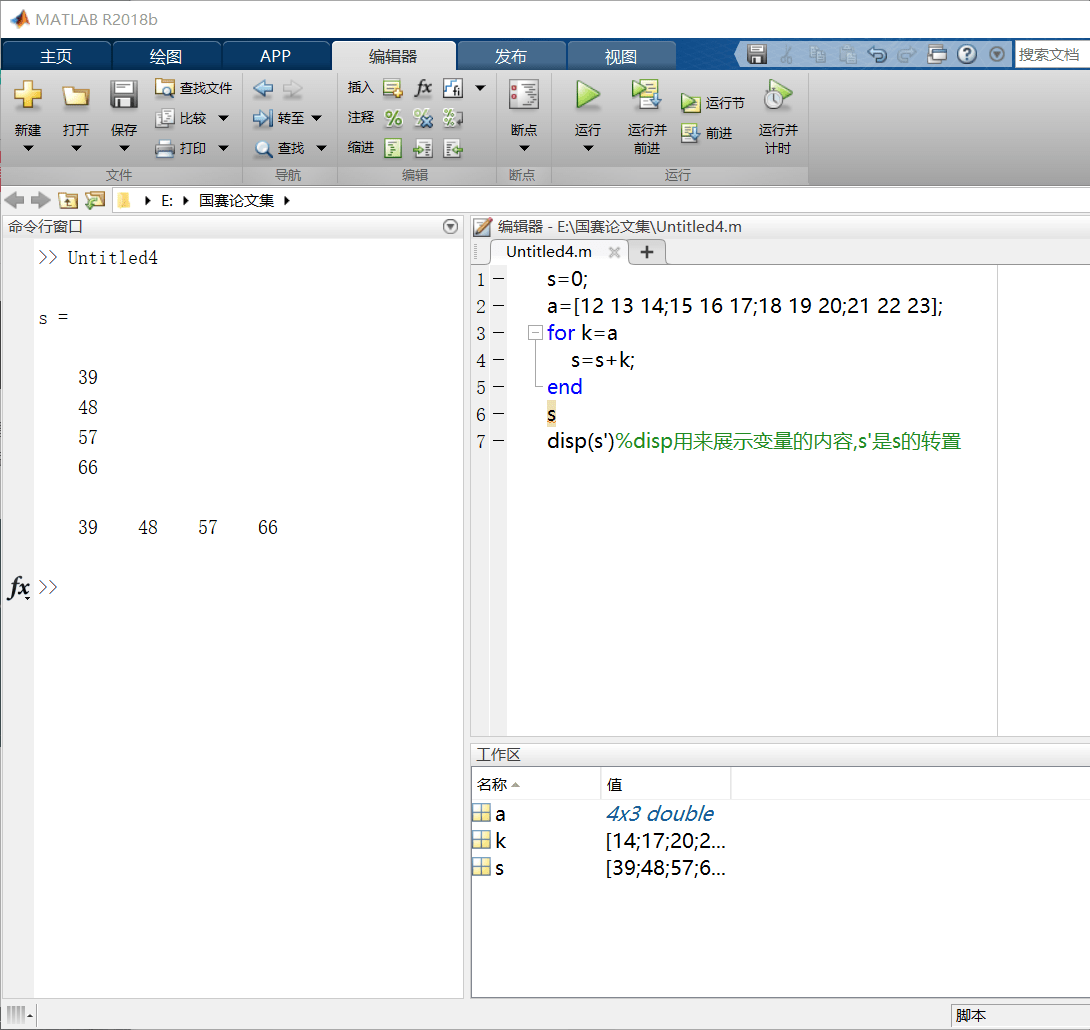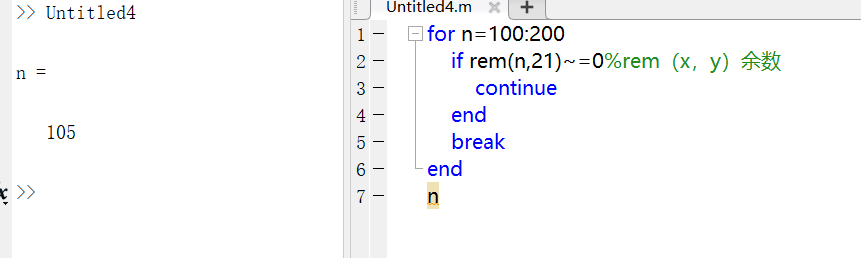# for循环

## 第一种格式

for 循环变量=初值：步长：终值

end``````y=0;
n=100;
for i=1:n
y=y+1/(2*i-1);
end
y
``````## 第二种格式

``````for 循环变量 = 矩阵表达式

end
``````# while循环

``````while 逻辑表达式

end
``````

``````sum=0;
i=1;
while i<=100
sum=sum+i;
i=i+1;
end
sum
``````# break和continue语句

break语句用于终止循环的执行。当在循环体内执行到该语句时候，程序将跳出循环，继续执行循环语句的下一个语句。
continue语句控制跳出循环中的某些语句。当循环体内执行到该语句时，程序将跳过循环体重所有剩下的语句，继续下一次循环。

# 循环的嵌套

（回溯算法可以减少嵌套）https://blog.csdn.net/diaomeijiao3430/article/details/101345151

（rem用的时候，可以搜“matlab中表示整除的函数”即可）

``````for i=1:6
for j=1:6
if i==j
A(i,j)=1;
elseif abs(i-j)==1%取绝对值
A(i,j)=2;
else
A(i,j)=0;
end
end
end
A
``````